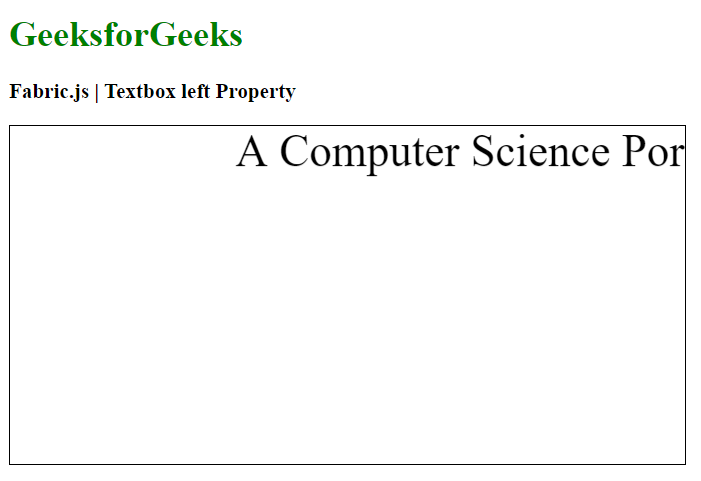# Fabric.js Textbox left Property

• Last Updated : 20 Jan, 2021

In this article, we are going to see how to set the left property of a Textbox canvas using FabricJS. The canvas Textbox means Textbox is movable and can be stretched according to requirement. Further, the Textbox can be customized when it comes to initial stroke color, height, width, fill color, or stroke width.

To make it possible, we are going to use a JavaScript library called FabricJS. After importing the library, we will create a canvas block in the body tag which will contain the Textbox. After this, we will initialize instances of Canvas and Textbox provided by FabricJS and set the value of the left property of a canvas, and render the Textbox on the Canvas as given in the below example.

Syntax:

```fabric.Textbox('text', {
left: number
});```

Parameters: This property accepts one parameter as mentioned above and described below:

• left: It specifies the left position of an object.

Example: This example uses FabricJS to set the left property on a canvas textbox.

## HTML

 ```<``html``>`` ` `<``head``>``    ``<``title``>``        ``Fabric.js | Textbox left Property``    ```` ` `    ````    ``<``script` `src``=``"https://cdnjs.cloudflare.com/ajax/libs/fabric.js/3.6.2/fabric.min.js"``>``    `````` ` `<``body``>``    ``<``h1` `style``=``"color: green;"``>``        ``GeeksforGeeks``    ```` ` `    ``<``h3``>``        ``Fabric.js | Textbox left Property``    ```` ` `    ``<``canvas` `id``=``"canvas"` `width``=``"600"` `height``=``"300"``        ``style``=``"border:1px solid #000000"``>``    ```` ` `    ``<``script``>`` ` `        ``// Initiate a Canvas instance ``        ``var canvas = new fabric.Canvas("canvas");`` ` `        ``// Create a new Textbox instance ``        ``var text = new fabric.Textbox(``            ``'A Computer Science Portal', {``            ``width: 500,``            ``left: 200``        ``});`` ` `        ``// Render the Textbox in canvas ``        ``canvas.add(text);``    `````` ` ``

Output:My Personal Notes arrow_drop_up